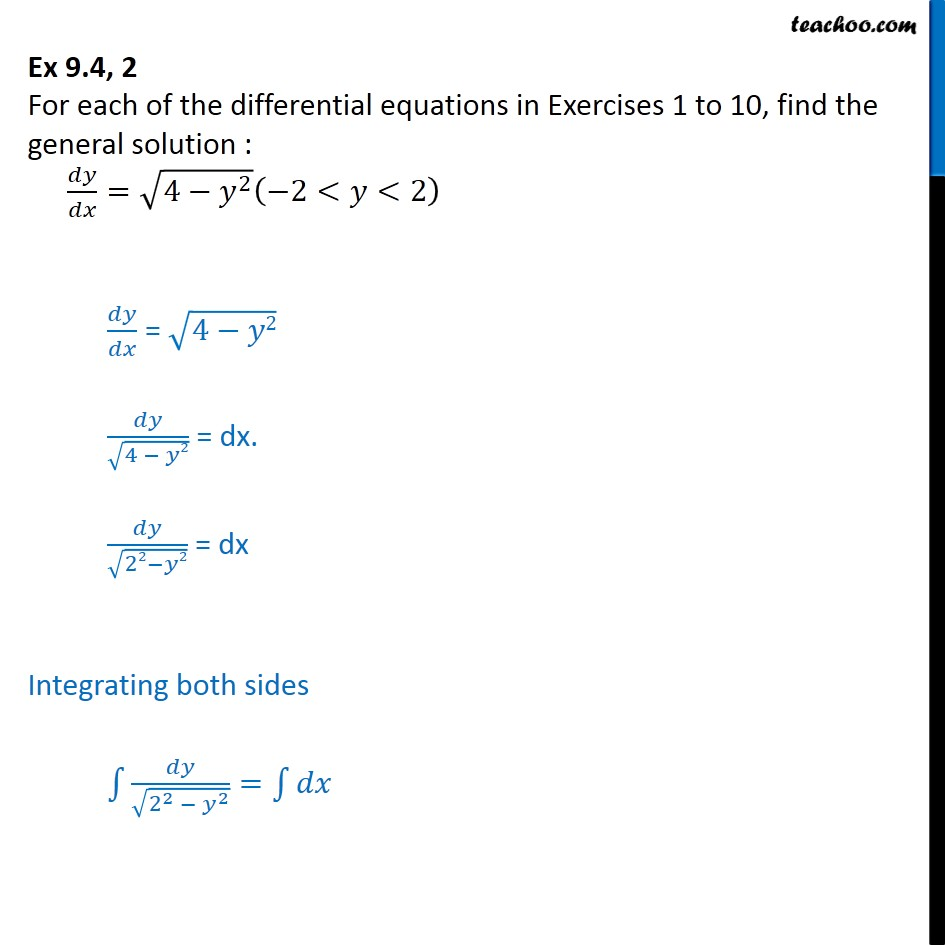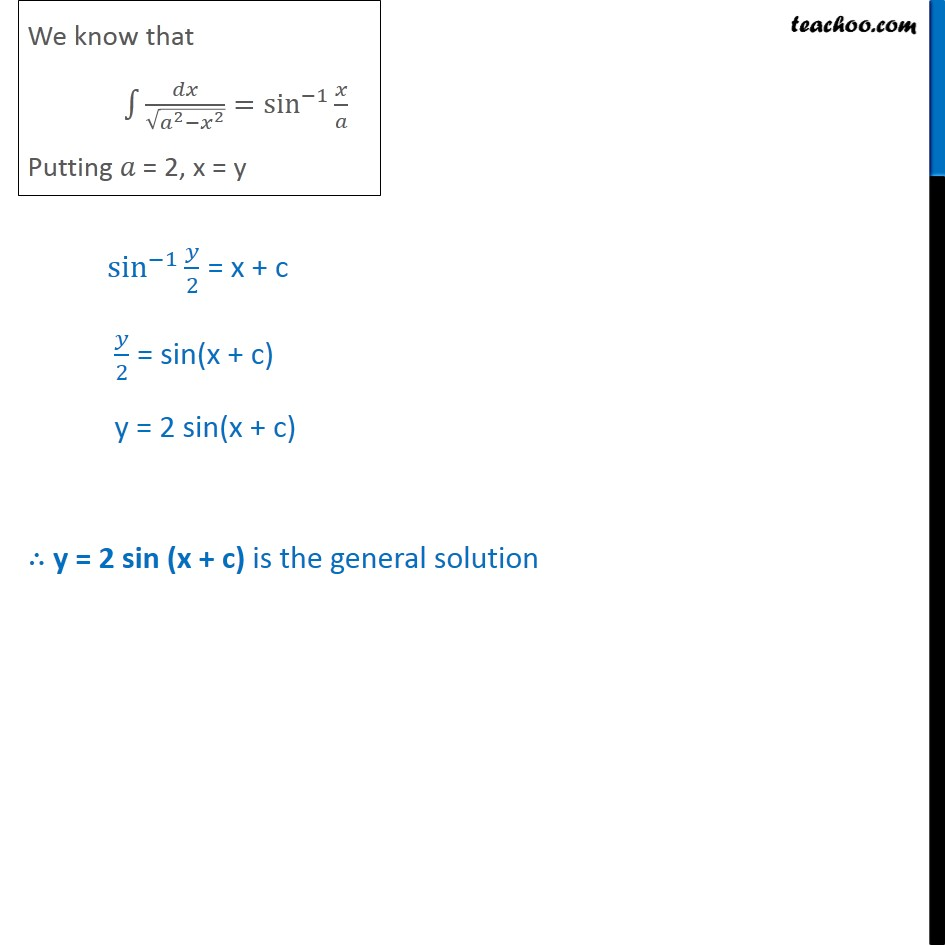Ex 9.3

Chapter 9 Class 12 Differential Equations
Serial order wiseLearn in your speed, with individual attention - Teachoo Maths 1-on-1 Class

### Transcript

Ex 9.3, 2 For each of the differential equations in Exercises 1 to 10, find the general solution : 𝑑𝑦﷮𝑑𝑥﷯= ﷮4− 𝑦﷮2﷯﷯ −2<𝑦<2﷯ 𝑑𝑦﷮𝑑𝑥﷯ = ﷮4−𝑦2﷯ 𝑑𝑦﷮ ﷮4 − 𝑦2﷯﷯ = dx. 𝑑𝑦﷮ ﷮22−𝑦2﷯﷯ = dx Integrating both sides ﷮﷮ 𝑑𝑦﷮ ﷮ 2﷮2﷯ − 𝑦﷮2﷯﷯﷯= ﷮﷮𝑑𝑥﷯﷯ sin﷮−1﷯﷮ 𝑦﷮2﷯﷯ = x + c 𝑦﷮2﷯ = sin(x + c) y = 2 sin(x + c) ∴ y = 2 sin (x + c) is the general solution